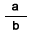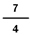# Mixed to fraction with number line models

## Learn or teach by designing your own mixed form to fraction form(improper) examples. All examples are modeled with number lines.

MIXED TO FRACTION WITH NUMBER LINE MODELS
SHOW COLOR
EXPLAIN
SHOW INPUT
WHOLE OR MIXED FORM TO FRACTION FORM

# INSTRUCTIONS

Mixed to Fraction with Number Line Models uses number lines to demonstrate renaming fractions from mixed form to fraction form.

Any whole number or mixed number can be written in fraction() form such as. Some textbooks call such a number where the numerator is equal to or greater than the denominator improper or top-heavy.

The very definition of a rational number uses the idea of fraction form. A rational number is any number that can be expressed as the quotient a/b of two integers, with the denominator b not equal to zero(0).

So a rational number is any number that can be written in fraction form as long as the denominator is not zero.

You can input any fraction with a value less than 7. The denominator must be less than 100. Press the <OK> button and the chosen fraction will appear in fraction form.

On the left is a <SHOW COLOR> check box. Uncheck the box to turn off the arrow and the red color on the number line. This will allow the learner to demonstrate the size of the fraction.

Uncheck the <EXPLAIN> check box to turn off the answer and the explanation. You can ask your students to complete the number sentence.

With <SHOW INPUT> unchecked, the fraction input boxes will act the same as password input boxes so students will not see the numbers you input.

With <EXPLAIN> and <SHOW INPUT> unchecked you can ask your students to write a number sentence that explains the picture.

With <EXPLAIN> and <SHOW COLOR> unchecked you can ask your students to shade the fraction and write in fraction(a/b) form.

Suggestions:

Demonstrate the numerator by counting each of the equal colored parts. So 1 13 will have 7 colored parts.

If you wish to see the fraction form of a whole number, type in a numerator that is a multiple of the denominator such as 164.

WINDOWS COMPUTERS

Windows users can select any part of the screen by right clicking and selecting "Take a screenshot". Adjust to fit mage you want. This copies the selection into Windows Clipboard™. The screen can then be pasted into Windows Paint™ or your favorite imaging program. Or you can select "Download" which will put the image into your files "Download" folder.